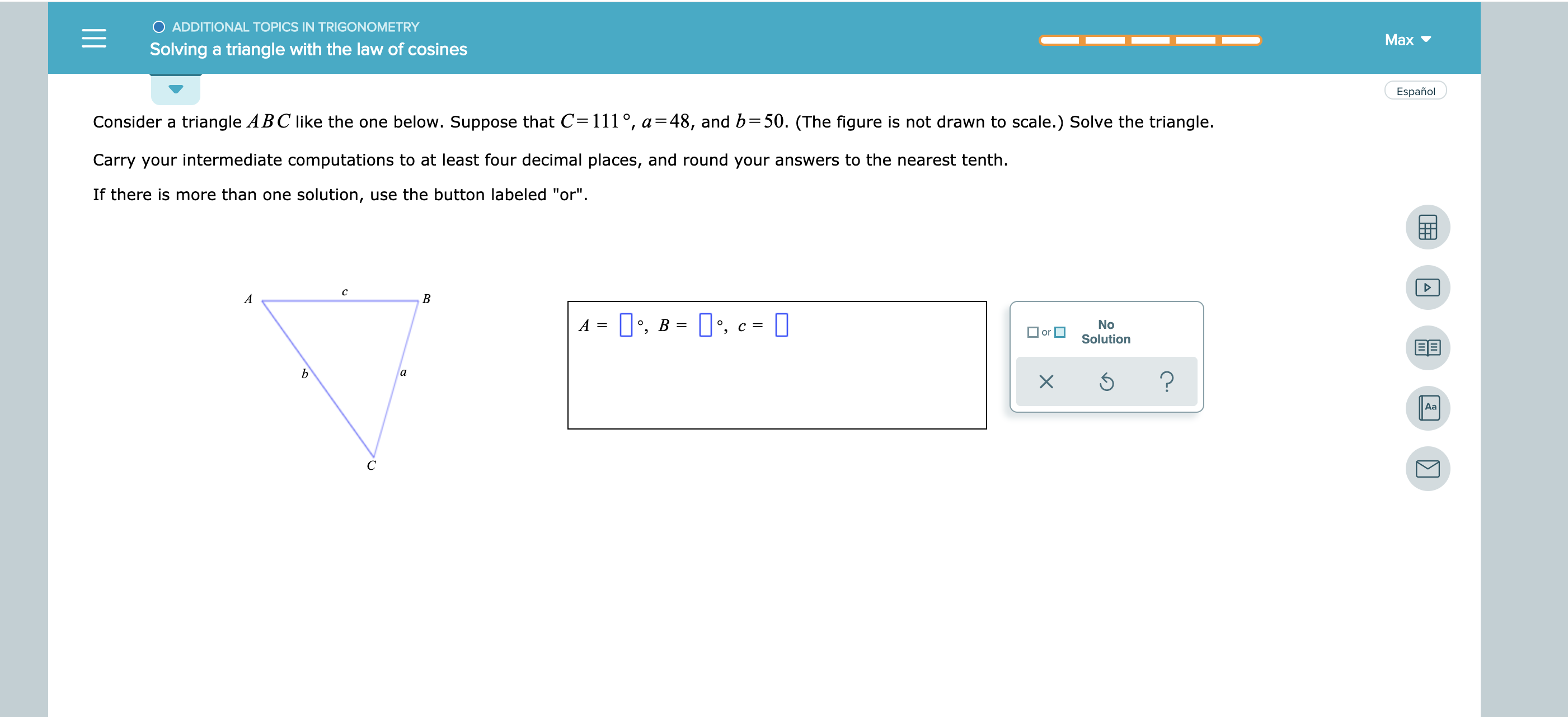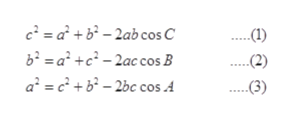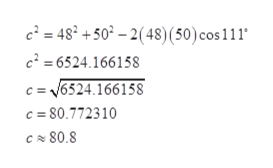# O ADDITIONAL TOPICS IN TRIGONOMETRYМахSolving a triangle with the law of cosinesEspañolConsider a triangle ABC like the one below. Suppose that C 111°, a=48, and b=50. (The figure is not drawn to scale.) Solve the triangle.Carry your intermediate computations to at least four decimal places, and round your answers to the nearest tenth.If there is more than one solution, use the button labeled "or"сABАNoorSolutionаXAaС

Question
3 views

see attachmenthelp_outlineImage TranscriptioncloseO ADDITIONAL TOPICS IN TRIGONOMETRY Мах Solving a triangle with the law of cosines Español Consider a triangle ABC like the one below. Suppose that C 111°, a=48, and b=50. (The figure is not drawn to scale.) Solve the triangle. Carry your intermediate computations to at least four decimal places, and round your answers to the nearest tenth. If there is more than one solution, use the button labeled "or" с A B А No or Solution а X Aa С fullscreen
check_circle

Step 1

Given,

Step 2

Apply Cosine Rule of angle,help_outlineImage Transcriptionclosec2= a +b-2ab cos C 62=a2 c2-2ac cos B a2c2b-2bc cos A -(1) -(2) .(3) fullscreen
Step 3

Plugging all value in...help_outlineImage Transcriptionclosec= 48 +50 -2( 48) (50) cos 111* c2=6524.166158 = v6524.166158 c = 80.772310 C 80.8 fullscreen

### Want to see the full answer?

See Solution

#### Want to see this answer and more?

Solutions are written by subject experts who are available 24/7. Questions are typically answered within 1 hour.*

See Solution
*Response times may vary by subject and question.
Tagged in

### Trigonometric Ratios